Courses

# Relational Algebra,Tuple Calculus And SQL (Basic Level)- 1

## 10 Questions MCQ Test Question Bank for GATE Computer Science Engineering | Relational Algebra,Tuple Calculus And SQL (Basic Level)- 1

Description
This mock test of Relational Algebra,Tuple Calculus And SQL (Basic Level)- 1 for Computer Science Engineering (CSE) helps you for every Computer Science Engineering (CSE) entrance exam. This contains 10 Multiple Choice Questions for Computer Science Engineering (CSE) Relational Algebra,Tuple Calculus And SQL (Basic Level)- 1 (mcq) to study with solutions a complete question bank. The solved questions answers in this Relational Algebra,Tuple Calculus And SQL (Basic Level)- 1 quiz give you a good mix of easy questions and tough questions. Computer Science Engineering (CSE) students definitely take this Relational Algebra,Tuple Calculus And SQL (Basic Level)- 1 exercise for a better result in the exam. You can find other Relational Algebra,Tuple Calculus And SQL (Basic Level)- 1 extra questions, long questions & short questions for Computer Science Engineering (CSE) on EduRev as well by searching above.
QUESTION: 1

### The relational algebra expression equivalent to the following expression: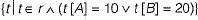is:

Solution:

The expression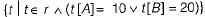can be represented in relational algebra as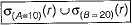It says that select those rows from relation r where A = 10 union where B = 20.
Hence option (b) is correct.

QUESTION: 2

Solution:
QUESTION: 3

### Which of the following statements is correct with respect to entity integrity?

Solution:

According to entity integrity primary key of relation should not contain null values.

QUESTION: 4

Which of the following operations is not part of the five basic set operations in relational algebra?

Solution:

Five primitive operators of Codd’s algebra are the selection, the projection the Cartesian product, the set union and the set difference.

QUESTION: 5

Which of the following relational algebraic operation is not a commutative operation?

Solution:
QUESTION: 6

Consider a banking database with the following relation with three attributes loans (br_name, loan_no, amount).
Find the appropriate query for the given statements below:
“Find the loan number for each loan of an amount greater than 20000”

Solution:

Loans (br_name, loan_no, amount)
For the query “find the loan number for each loan of an amount greater than 20000”.
We have to check for each loan to find a loan number which is greater than 20,000.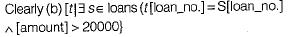Hence (b) is the correct option.

QUESTION: 7

Let R(a, b, c) and S(d, e, f) be two relations in which d is the foreign key of S that refers to the primary key of R. Consider the following four operations R and S
1. Insert into R
2. Insert into S
3. Delete from R
4. Delete from S
Which of the following is true about the referential integrity constraint above?

Solution:

Referential integrity constraint: In relational model, two relation are related to each other over the basis of attributes, Every value of referencing attribute must be null or be available in the referenced attribute.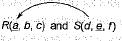Here d is the foreign key of S that refers to the primary key of R.
1. Insert into R will not cause any violation.
2. Insert into S may cause violation because for each entry in ‘S ’ it must be. in ‘R ’ .
3. Delete from R may cause violation because for the deleted entry in R there may be referenced entry in the reIation S.
4. Delete from S will not cause any violation.
Hence (d) is the correct option.

QUESTION: 8

Let r and s be two relations over the relation schemes R and S respectively, and let A be an attribute in R. Then the relational algebra expression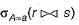is always equal to

Solution:

The relational algebra expression: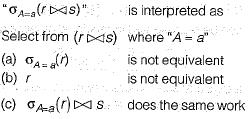Hence (c) is the required option.

QUESTION: 9

Join Selectivity of a relation R in a natural join with a relation S is the _____

Solution:
QUESTION: 10

Which of the following is wrong?

Solution: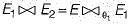is not equivalent because θ join is not cumulative hence the entries in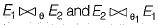may not be same. Hence option (d) is correct.